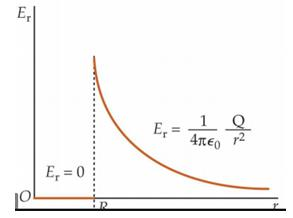Courses

# Test: Electric Flux & Gauss Law

## 20 Questions MCQ Test Physics For JEE | Test: Electric Flux & Gauss Law

Description
This mock test of Test: Electric Flux & Gauss Law for JEE helps you for every JEE entrance exam. This contains 20 Multiple Choice Questions for JEE Test: Electric Flux & Gauss Law (mcq) to study with solutions a complete question bank. The solved questions answers in this Test: Electric Flux & Gauss Law quiz give you a good mix of easy questions and tough questions. JEE students definitely take this Test: Electric Flux & Gauss Law exercise for a better result in the exam. You can find other Test: Electric Flux & Gauss Law extra questions, long questions & short questions for JEE on EduRev as well by searching above.
QUESTION: 1

### If the electric field is given by 26i – k. Calculate the electric flux through a surface of area 20 units lying in x-y plane.

Solution:

► Electric field (E) = 26i - k
► A = 20k
Electric flux = electric field.area
= E.A
= (26i - k)*(20k)
= (26*0 -1)*20
= 20 units

QUESTION: 2

### A point charge of 2.0 μC is at the center of a cubic Gaussian surface 9.0 cm on edge. What is the net electric flux through the surface?

Solution:

Net electric flux (ΦNet) through the cubic surface is given by,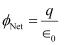Where, ∈0 = Permittivity of free space

= 8.854 × 10−12 N−1C2 m−2

q = Net charge contained inside the cube = 2.0 μC = 2 × 10−6 C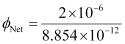= 2.2 × 105 N m2 C−1

The net electric flux through the surface is 2.2 ×105 N m2C−1.

QUESTION: 3

### Electric flux through an area dA for an electric field E is given by​:

Solution: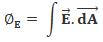For constant electric field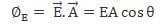QUESTION: 4

A point charge causes an electric flux of −1.0×103 Nm2/C to pass through a spherical Gaussian surface of 10.0 cm radius centered on the charge.
(a) If the radius of the Gaussian surface were doubled, how much flux would pass through the surface?
(b) What is the value of the point charge?

Solution:

Electric flux is given by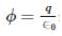since amount of charge not depends on size and shape so by making radius <  double the amount of charge remain same so electric flux remain same.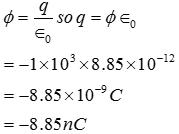QUESTION: 5

Choose the correct statement:

Solution:

By definition of Solid Angle,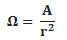Where, Ω is represented in Steradians.

QUESTION: 6

Electric flux is a _______ quantity.

Solution:

Electric flux is a scalar quantityElectric Flux is dot/scalar product of magnetic field vector and area vector. And the result of a dot/scalar quantity is always a scalar quantity. So electric flux is also a scalar quantity

QUESTION: 7

If the electric field is given by 6i+3j+4k calculate the electric flux through a surface of area 20 units lying in y-z plane.

Solution:

► Area = 20i since it is in the Y-Z plane
► E = 6i + 3j + 4k
► E.S = Flux
= 20*6 = 120 units

QUESTION: 8

A uniform line charge with linear density λ lies along the y-axis. What flux crosses a spherical surface centred at the origin with r = R?

Solution:

Line charge density = λ
Now,  λdl=dθ
For total charge enclosed by radius R, 0R Q = 0R λdl
Qinc = λR
Now, ϕ = Qinco    {from Gauss law}
= λR/ε0

QUESTION: 9

Dimensional formula of ØE is​:

Solution:

Flux = E. A
⇒ F = Eq
⇒ E= F/q= N/C
⇒ Flux= N/C × m²
= Nm2C-1

QUESTION: 10

Electric flux density is a function of _______.

Solution:

Electric flux density is the charge per unit area. Hence it is a function of charge and not any of the other values.

QUESTION: 11

Which of the following correctly states Gauss law?

Solution:

The electric flux passing through any closed surface is equal to the total charge enclosed by that surface. In other words, electric flux per unit volume leaving a point (vanishing small volume), is equal to the volume charge density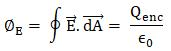QUESTION: 12

Electric flux of a surface is maximum, when

Solution:

By definition,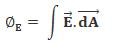For maximum, E.dA must be maximum. This is possible when E || dA

QUESTION: 13

The Gaussian surface for a point charge will be

Solution:

A point charge is single dimensional. The three dimensional imaginary enclosed surface of a point charge will be sphere.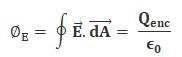QUESTION: 14

At what point is the electric field intensity due to a uniformly charged spherical shell is maximum?

Solution:

By Gauss Law,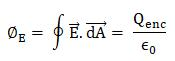Electric Field due to spherical shell shows the following behavior: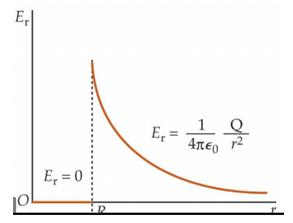QUESTION: 15

A charge q is placed at the centre of the open end of cylindrical vessel whose height is equal to its radius. The electric flux of electric field of charge q through the surface of the vessel is

Solution: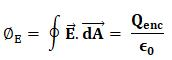We can assume a similar vessel, such that the charge lies at the interfaces of both the vessels. Now, by Gauss law, all the electric flux must pass through these two vessels. As they are kept symmetrically with respect to the charge equal amount of flux would pass through them

From both vessels,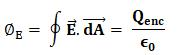For each vessel,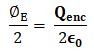QUESTION: 16

The charge enclosed by a spherical Gaussian surface is 8.85 X 10-8 C. What will be the electric flux through the Surface?

Solution:

We know that the flux linked with a Gaussian surface is given as:

Φ = E.ds = q/ε0

Here,

q = 8.85 x 10-8 C

ε0 = 8.85 x 10-12 F/m

So,

Φ = 8.85x10-8/ 8.85x10-12

Thus, flux will be

Φ = 10-4 Nm2/C

QUESTION: 17

If the electric field is given by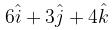calculate the electric flux through as surface of area 10 units lying in y-z plane.

Solution: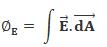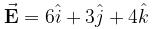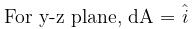Hence,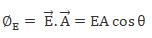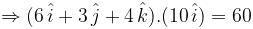QUESTION: 18

The flux associated with a spherical surface is 104 N m2C-1.  What will be the flux, if the radius of the spherical shell is doubled?

Solution:

If the radius of the spherical shell is double then also flux associated with spherical surface remain unchanged because the charge enclosed in system always remains unchanged.

QUESTION: 19

The Gaussian surface for a line charge will be

Solution:

A line charge can be visualized as a rod of electric charges. The three dimensional imaginary enclosed surface of a rod can be a cylinder.

QUESTION: 20

The electric field intensity due to a sphere (solid or hollow) at an external point varies as:

Solution:

We calculate this using spherical symmtery in Gauss Law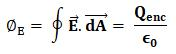For spherical Shell,